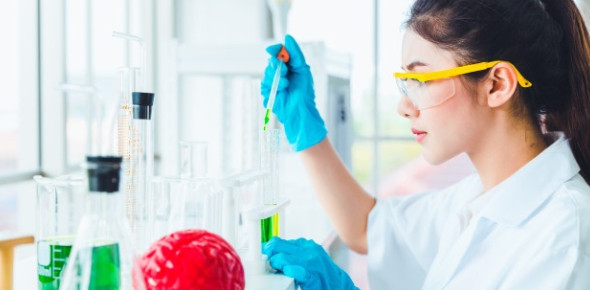The Ultimate Chemistry Exams Prep Test

65 Questions | Total Attempts: 7887SettingsIn chemistry classes, we get to discuss a lot about liquid and how to form a solution. With the midterms just around the corner, it is becoming more critical to ensure that you are up to date with all covered in class so far. Below is a The Ultimate Chemistry Exams Prep Test designed to refresh your memory. Give it a shot!

• 1.
Convert 20g to kg.
• A.

2000kg

• B.

20000kg

• C.

0.20kg

• D.

0.020kg

• 2.
Convert 23ml to liters.
• A.

0.023L

• B.

230L

• C.

230000L

• D.

23000L

• 3.
Convert 18km to mm.
• A.

18000mm

• B.

0.0018mm

• C.

18000000mm

• D.

1.80mm

• 4.
Convert 88kg to mg.
• A.

8.8mg

• B.

88000mg

• C.

0.0000088mg

• D.

88000000mg

• 5.
Convert 23.6cm to mm.
• A.

2.36mm

• B.

236mm

• C.

0.236mm

• D.

0.0236mm

• 6.
Define matter:
• A.

The smallest particle of an element

• B.

The smallest particles on earth

• C.

Anything that has mass and occupies space

• D.

A positively charge particle

• 7.
Name the three states of matter:
• A.

Protons, neutrons, and electrons

• B.

Metals, non-metals, and metalloids

• C.

Solid, liqui, and gas

• D.

Freezing, melting, and evaporation

• 8.
What instruments do we use to measure volume?
• A.

Thermometer

• B.

• C.

Scale

• D.

Ruler

• 9.
What instruments do we use to measure the mass:
• A.

• B.

Thermometer

• C.

Beaker

• D.

Scale

• 10.
What instruments do we use to measure density?
• A.

Scale and thermometer

• B.

Scale and stop watch

• C.

• D.

• 11.
What SI unit is used to measure volume
• A.

Grams

• B.

Kilometer

• C.

Liters

• D.

Degree celcius

• 12.
Properties that cannot be observed with the five senses are called:
• A.

Sub-microscopic properties

• B.

Macroscopic properties

• C.

Physical properties

• D.

Biological properties

• 13.
A substance consisting of two elements chemically combined is known as:
• A.

Mixture

• B.

Solution

• C.

Element

• D.

Compound

• 14.
A mixture that separate into two visible phases is known as:
• A.

Solution

• B.

Heterogeneous mixture

• C.

Homogeousmixture

• D.

Compound

• 15.
A positive charge particle found in the nucleus is called:
• A.

Neutron

• B.

Proton

• C.

Electron

• D.

Atom

• 16.
A change from liquid to solid is called:
• A.

Melting

• B.

Boiling

• C.

Freezing

• D.

Condensation

• 17.
A change from liquid to gas is known as:
• A.

Condensation

• B.

Melting

• C.

Sublimation

• D.

Evaporation

• 18.
A change from gas to liquid is called:
• A.

Sublimation

• B.

Evaporation

• C.

Freezing

• D.

Condensation

• 19.
A mixture of sand and iron particles can be separated by:
• A.

Distillation

• B.

Magnetism

• C.

Decantation

• D.

Filtration

• 20.
A mixture of salt and water can be separated by:
• A.

condensation

• B.

Filtration

• C.

Crystalization

• D.

Distillation

• 21.
A mixture of sand and water can be separated by:
• A.

Filtration

• B.

Evaporation

• C.

Distillation

• D.

Crystalization

• 22.
A mixture of peroxide and water can be separated by:
• A.

Evaporation

• B.

Sublimation

• C.

Decantation

• D.

Distillation

• 23.
Burning wood is an example of:
• A.

Physical change

• B.

Chemical change

• 24.
Baking cake is classified as:
• A.

Physical change

• B.

Chemical change

• 25.
Melting candle wax is classified as:
• A.

Physical change

• B.

Chemical change

Related TopicsBack to top